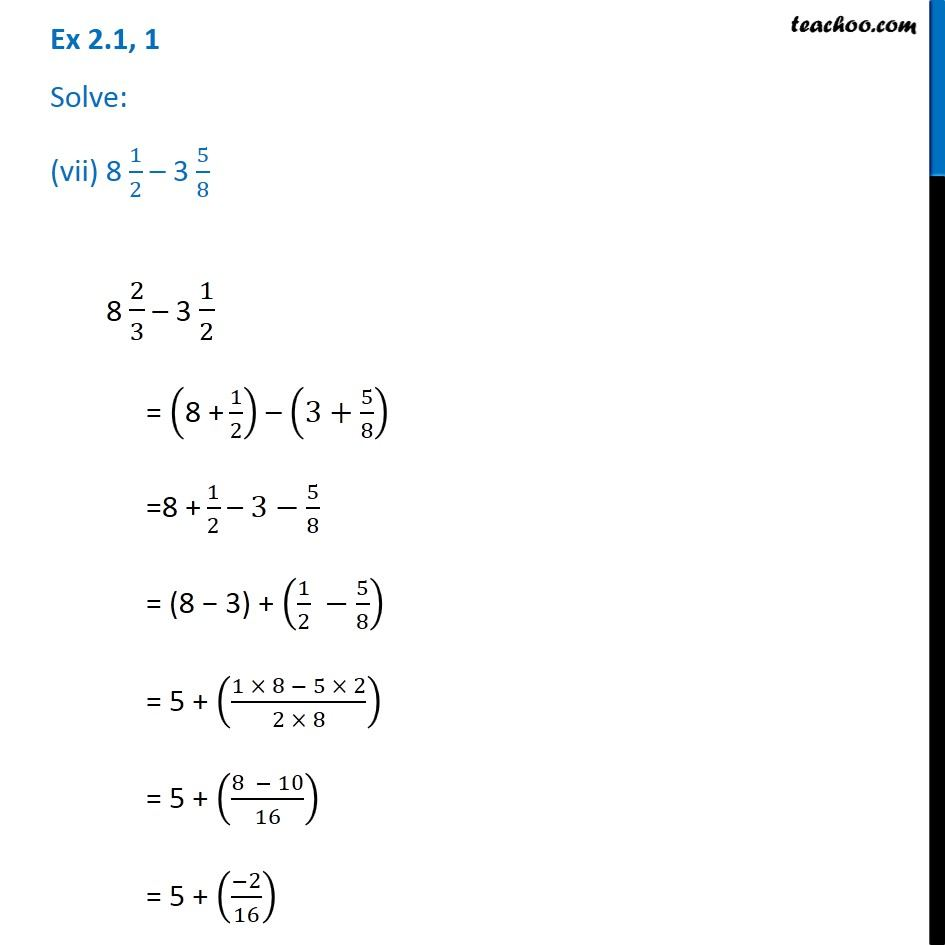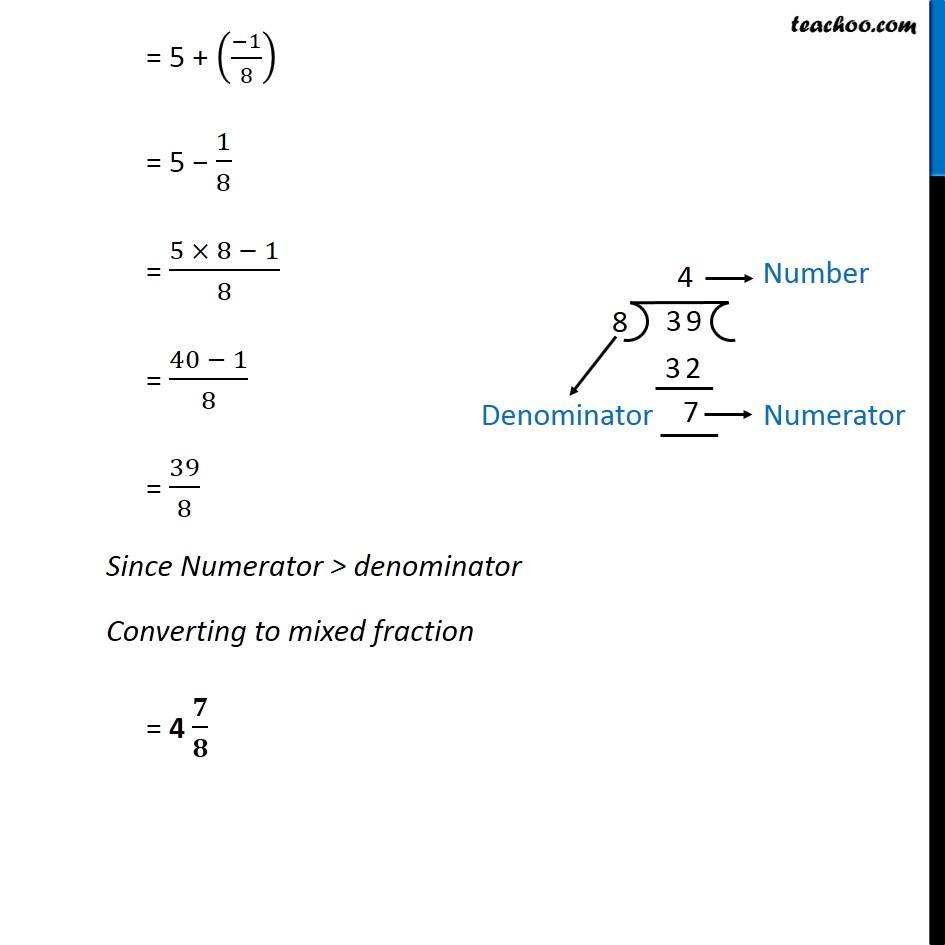Chapter 2 Class 7 Fractions and Decimals
Serial order wiseLearn in your speed, with individual attention - Teachoo Maths 1-on-1 Class

### Transcript

Question 1 Solve: (vii) 8 1/2 – 3 5/8 8 2/3 – 3 1/2 = ("8 +" 1/2) – (3+5/8) ="8 +" 1/2 – 3−5/8 = (8 − 3) + (1/2 −5/8) = 5 + ((1 × 8 − 5 × 2)/(2 × 8)) = 5 + ((8 − 10)/16) = 5 + ((−2)/16) = 5 + ((−1)/8) = 5 − 1/8 = (5 × 8 − 1)/8 = (40 − 1)/8 = 39/8 Since Numerator > denominator Converting to mixed fraction = 4 𝟕/𝟖